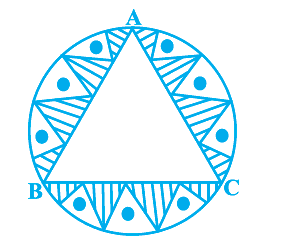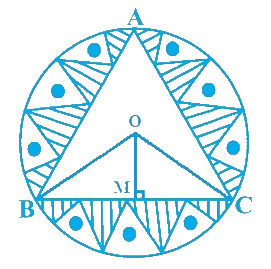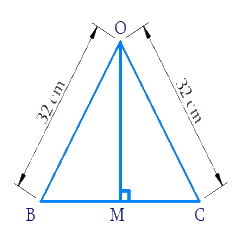# Ex.12.3 Q6 Areas Related to Circles Solution - NCERT Maths Class 10

Go back to  'Ex.12.3'

## Question

In a circular table cover of radius $$\text{32 cm,}$$ a design is formed leaving an equilateral triangle $$ABC$$ in the middle as shown in the given figure. Find the area of the design.Video Solution
Areas Related To Circles
Ex 12.3 | Question 6

## Text Solution

What is known?

A circular table cover of radius $$\text{= 32 cm,}$$ a design is formed leaving an equilateral triangle $$ABC$$ in the middle as shown in figure.

What is unknown?

The area of the design.

Reasoning:Mark $$O$$ as centre of the circle. Join $$BO$$ and $$CO.$$

Since ,we know that equal chords of a circle subtends equal angles at the center and all sides of an equilateral triangle are equal.

$$\therefore \;$$ Each side of $$\Delta$$ will subtend equal angles at centre

\begin{align}\therefore \angle BOC = \frac{{{{360}^\circ }}}{3}\,\, = {120^\circ }\end{align}Consider$$\Delta BOC$$

Drop a perpendicular from $$OM$$ to $$BC$$

Since we know perpendicular from the center of circle to a chord bisects it

$$\Rightarrow \quad BM = MC$$

$$OB = OC$$ (radii) and $$OM = OM$$(common)

$$\therefore \Delta {OBM} \cong \Delta {OCM}$$ (by SSS congruency)

$$\Rightarrow \angle BOM\, =\,\angle COM$$ (Corresponding Parts of Congruent Triangles are Congrue (CPCTC))

$$\therefore \,2\angle {BOM}=\angle {BOC}={{120}^{\circ }}$$

\begin{align}\angle {BOM}&=\frac{{{120}^{\circ }}}{2}\\&={{60}^{\circ }} \\ \frac{{BM}}{{BO}}&=\sin \,{{60}^{\circ }}\\&=\frac{\sqrt{3}}{2} \\{BM}&=\frac{\sqrt{3}}{2}\times {BO}\\&=\frac{\sqrt{3}}{2}\times 32\\&=16\sqrt{3}\\ \quad {BC}&=2 {BM}\\&=32\sqrt{3}\,cm \\ \end{align}

Using formula of area of equilateral $$\Delta$$

\begin{align} = \frac{{\sqrt 3 }}{4}{({\text{side}})^2}\end{align}

We can find area of $$\Delta {ABC}$$ since a side $$BC$$ of $$\Delta$$ is known.

Visually from figure it’s clear

Area of the design $$=$$  Area of circle $$-$$  Area of $$\Delta ABC$$

\begin{align}= \pi {r^2} - \frac{{\sqrt 3 }}{4}{({BC})^2}\end{align}

This can be solved with ease as both the radius of the circle and $$BC$$ are known.

Steps:Let the center of the circle be $$O.$$ Join $$BO$$ and $$CO.$$

Since equal chords of a circle subtend equal angles as its centre.

$$\therefore \;$$ Sides $$AB, BC$$ and $$AC$$ of $$\Delta ABC$$ will subtend equal angles at the centre of circle

\begin{align}\therefore \angle {BOC} = \frac{{{{360}^\circ }}}{3} = {120^\circ }\end{align}

Draw $${{OM}} \bot {{BC}}$$

In $$\Delta BOM\ {\rm and} \Delta COM$$

$$BO = CO$$ (radii circle)

$$OM = OM$$ (common)

$$BM = CM$$.... (perpendicular drawn from the center of the circle to a chord bisects it)

$$\Delta {BOM} \cong \Delta {COM}$$ (By SSS Congruency)

$$\therefore \;\angle {BOM} = \angle {COM} \dots \dots(2)$$(CPCT)

From figure

\begin{align}\angle {BOM} + \angle {COM} &= \angle {BOC}\\2\angle {BOM} &= \angle {BOC}\\ &= {120^ \circ }\\&\left( {{\text{Using}}\,{\text{ }}\left( 2 \right)} \right)\\\angle {BOM} &= \frac{{{{120}^ \circ }}}{2} = {60^ \circ }\end{align}

In $$\Delta {BOM}$$

\begin{align}\frac{{BM}}{{BO}} &= \sin \,{60^ \circ } = \frac{{\sqrt 3 }}{2}\\\therefore {BM} &\!=\! \frac{{\sqrt 3 }}{2}{BO}\!=\!\frac{{\sqrt 3 }}{2}\!\!\times\!32\!=\!16\sqrt 3 \\{\text{BC}} &= {\text{BM}} + {\text{CM}} = 2{\text{BM}}\\&\qquad({\text{using}}(1))\\\,\,\,\,\,\,\,\, &= 2 \times 16\sqrt 3 \\\,\,\,\,\,\,\,\, &= 32\sqrt 3 \end{align}

Radius of circle $$(r) =\text{ 32 cm}$$

From figure, we observe

Area of design $$=$$ Area of circle $$-$$ Area of $$\Delta {ABC}$$

\begin{align} &={\pi {r^2} - \frac{{\sqrt 3 }}{4}{{(BC)}^2}}\\ &={\pi {{(32)}^2} - \frac{{\sqrt 3 }}{4}{{(32\sqrt 3 )}^2}}\\ &= {\pi {{(32)}^2} - \frac{{\sqrt 3 }}{4}{{(32)}^2} \times 3}\\ &={\frac{{22528}}{7} \times 1024 - 32 \times 24\sqrt 3 }\\ &= {\frac{{22528}}{7} - 768\sqrt 3}\end{align}

Area of Design \begin{align} = \left( {\frac{{22528}}{7} - 768\sqrt 3 } \right)\rm{cm^2}\end{align}

Learn from the best math teachers and top your exams

• Live one on one classroom and doubt clearing
• Practice worksheets in and after class for conceptual clarity
• Personalized curriculum to keep up with school